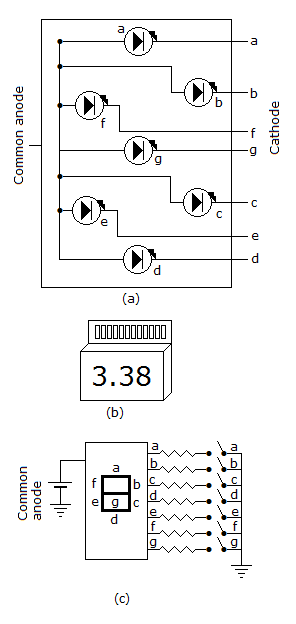# Electronics and Communication Engineering - Digital Electronics

6.

The circuit of the given figure is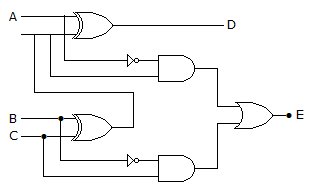A. full adder B. full subtractor C. shift register D. decade counter

Explanation: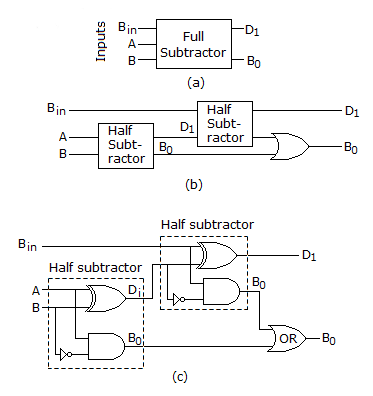7.

 A. 2 bits B. 3 bits C. 4 bits D. 2 or 3 bits

Explanation: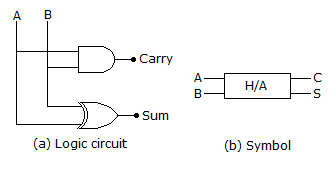8.

The no. of comparators required in a 3 bit comparator type ADC is

 A. 2 B. 3 C. 7 D. 8

Explanation:

Number of comparators = 2n - 1 = 23 - 1 = 7.

9.

If the ladder reference voltage is 2 V, then minimum comparator resolution required is

 A. 0.125 V B. 1.25 V C. 12.5 V D. 0

Explanation: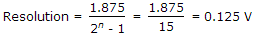.

10.

In a 7 segment LED display, the minimum number of segments is activated when the input decimal number is

 A. 0 B. 1 C. 2 D. 3

Explanation:

Only 2 segments are lit as seen in